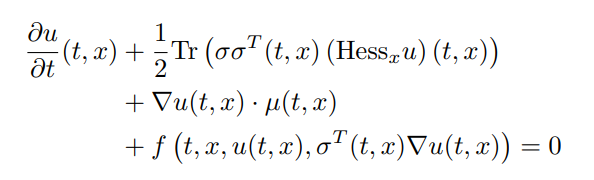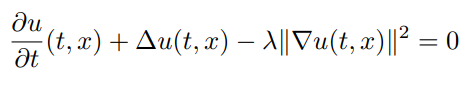Solves ordinary differential equations and partial differential equations using neural nets
Julia TeXFetching latest commit…
Cannot retrieve the latest commit at this time.
Type Name Latest commit message Commit time
Failed to load latest commit information..github/workflowssrctest.codecov.yml.gitignore.travis.ymlCITATION.bibLICENSE.mdProject.tomlREADME.mdappveyor.yml

# NeuralNetDiffEq

The repository is for the development of neural network solvers of differential equations. It utilizes techniques like neural stochastic differential equations to make it practical to solve high dimensional PDEs of the form:Additionally it utilizes neural networks as universal function approximators to solve ODEs. These are techniques of a field becoming known as Scientific Machine Learning (Scientific ML), encapsulated in a maintained repository.

# Examples

## Solving the 100 dimensional Black-Scholes-Barenblatt Equation

In this example we will solve a Black-Scholes-Barenblatt equation of 100 dimensions. The Black-Scholes-Barenblatt equation is a nonlinear extension to the Black-Scholes equation which models uncertain volatility and interest rates derived from the Black-Scholes equation. This model results in a nonlinear PDE whose dimension is the number of assets in the portfolio. The PDE is of the form:To solve it using the `TerminalPDEProblem`, we write:

```d = 100 # number of dimensions
X0 = repeat([1.0f0, 0.5f0], div(d,2)) # initial value of stochastic state
tspan = (0.0f0,1.0f0)
r = 0.05f0
sigma = 0.4f0
f(X,u,σᵀ∇u,p,t) = r * (u - sum(X.*σᵀ∇u))
g(X) = sum(X.^2)
μ(X,p,t) = zero(X) #Vector d x 1
σ(X,p,t) = Diagonal(sigma*X.data) #Matrix d x d
prob = TerminalPDEProblem(g, f, μ, σ, X0, tspan)```

As described in the API docs, we now need to define our `NNPDENS` algorithm by giving it the Flux.jl chains we want it to use for the neural networks. `u0` needs to be a `d` dimensional -> 1 dimensional chain, while `σᵀ∇u` needs to be `d+1` dimensional to `d` dimensions. Thus we define the following:

```hls  = 10 + d #hide layer size
u0 = Flux.Chain(Dense(d,hls,relu),
Dense(hls,hls,relu),
Dense(hls,1))
σᵀ∇u = Flux.Chain(Dense(d+1,hls,relu),
Dense(hls,hls,relu),
Dense(hls,hls,relu),
Dense(hls,d))
pdealg = NNPDENS(u0, σᵀ∇u, opt=opt)```

And now we solve the PDE. Here we say we want to solve the underlying neural SDE using the Euler-Maruyama SDE solver with our chosen `dt=0.2`, do at most 150 iterations of the optimizer, 100 SDE solves per loss evaluation (for averaging), and stop if the loss ever goes below `1f-6`.

```ans = solve(prob, pdealg, verbose=true, maxiters=150, trajectories=100,
alg=EM(), dt=0.2, pabstol = 1f-6)```

## Solving a 100 dimensional Hamilton-Jacobi-Bellman Equation

In this example we will solve a Hamilton-Jacobi-Bellman equation of 100 dimensions. The Hamilton-Jacobi-Bellman equation is the solution to a stochastic optimal control problem. Here, we choose to solve the classical Linear Quadratic Gaussian (LQG) control problem of 100 dimensions, which is governed by the SDE `dX_t = 2sqrt(λ)c_t dt + sqrt(2)dW_t` where `c_t` is a control process. The solution to the optimal control is given by a PDE of the form:with terminating condition `g(X) = log(0.5f0 + 0.5f0*sum(X.^2))`. To solve it using the `TerminalPDEProblem`, we write:

```d = 100 # number of dimensions
X0 = fill(0.0f0,d) # initial value of stochastic control process
tspan = (0.0f0, 1.0f0)
λ = 1.0f0

g(X) = log(0.5f0 + 0.5f0*sum(X.^2))
f(X,u,σᵀ∇u,p,t) = -λ*sum(σᵀ∇u.^2)
μ(X,p,t) = zero(X)  #Vector d x 1 λ
σ(X,p,t) = Diagonal(sqrt(2.0f0)*ones(Float32,d)) #Matrix d x d
prob = TerminalPDEProblem(g, f, μ, σ, X0, tspan)```

As described in the API docs, we now need to define our `NNPDENS` algorithm by giving it the Flux.jl chains we want it to use for the neural networks. `u0` needs to be a `d` dimensional -> 1 dimensional chain, while `σᵀ∇u` needs to be `d+1` dimensional to `d` dimensions. Thus we define the following:

```hls = 10 + d #hidden layer size
#sub-neural network approximating solutions at the desired point
u0 = Flux.Chain(Dense(d,hls,relu),
Dense(hls,hls,relu),
Dense(hls,1))
# sub-neural network approximating the spatial gradients at time point
σᵀ∇u = Flux.Chain(Dense(d+1,hls,relu),
Dense(hls,hls,relu),
Dense(hls,hls,relu),
Dense(hls,d))
pdealg = NNPDENS(u0, σᵀ∇u, opt=opt)```

And now we solve the PDE. Here we say we want to solve the underlying neural SDE using the Euler-Maruyama SDE solver with our chosen `dt=0.2`, do at most 100 iterations of the optimizer, 100 SDE solves per loss evaluation (for averaging), and stop if the loss ever goes below `1f-2`.

```@time ans = solve(prob, pdealg, verbose=true, maxiters=100, trajectories=100,
alg=EM(), dt=0.2, pabstol = 1f-2)
```

# API Documentation

## Solving High Dimensional PDEs with Neural Networks

To solve high dimensional PDEs, first one should describe the PDE in terms of the `TerminalPDEProblem` with constructor:

`TerminalPDEProblem(g,f,μ,σ,X0,tspan,p=nothing)`

which describes the semilinear parabolic PDE of the form:with terminating condition `u(tspan,x) = g(x)`. These methods solve the PDE in reverse, satisfying the terminal equation and giving a point estimate at `u(tspan,X0)`. The dimensionality of the PDE is determined by the choice of `X0`, which is the initial stochastic state.

To solve this PDE problem, there exists two algorithms:

• `NNPDENS(u0,σᵀ∇u;opt=Flux.ADAM(0.1))`: Uses a neural stochastic differential equation which is then solved by the methods available in DifferentialEquations.jl The `alg` keyword is required for specifying the SDE solver algorithm that will be used on the internal SDE. All of the other keyword arguments are passed to the SDE solver.
• `NNPDEHan(u0,σᵀ∇u;opt=Flux.ADAM(0.1))`: Uses the stochastic RNN algorithm from Han. Only applicable when `μ` and `σ` result in a non-stiff SDE where low order non-adaptive time stepping is applicable.

Here, `u0` is a Flux.jl chain with `d` dimensional input and 1 dimensional output. For `NNPDEHan`, `σᵀ∇u` is an array of `M` chains with `d` dimensional input and `d` dimensional output, where `M` is the total number of timesteps. For `NNPDENS` it is a `d+1` dimensional input (where the final value is time) and `d` dimensional output. `opt` is a Flux.jl optimizer.

Each of these methods has a special keyword argument `pabstol` which specifies an absolute tolerance on the PDE's solution, and will exit early if the loss reaches this value. Its defualt value is `1f-6`.

## Solving ODEs with Neural Networks

For ODEs, see the DifferentialEquations.jl documentation for the `nnode(chain,opt=ADAM(0.1))` algorithm, which takes in a Flux.jl chain and optimizer to solve an ODE. This method is not particularly efficient, but is parallel. It is based on the work of:

Lagaris, Isaac E., Aristidis Likas, and Dimitrios I. Fotiadis. "Artificial neural networks for solving ordinary and partial differential equations." IEEE Transactions on Neural Networks 9, no. 5 (1998): 987-1000.

You can’t perform that action at this time.